# Laplace theorem

(diff) ← Older revision | Latest revision (diff) | Newer revision → (diff)
Jump to: navigation, search

Laplace's theorem on determinants. See Cofactor.

Laplace's theorem on the approximation of the binomial distribution by the normal distribution. This is the first version of the central limit theorem of probability theory: Ifis the number of "successes" inBernoulli trials with probability of success,, then, as, for any real numbersand() one has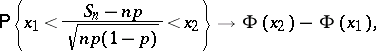(*)

whereis the distribution function of the standard normal law.

The local Laplace theorem has independent significance: For the probability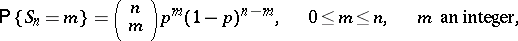one haswhere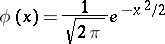is the density of the standard normal distribution and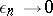asuniformly for allfor which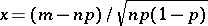belongs to some finite interval.

In its general form the theorem was proved by P.S. Laplace . The special case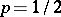of the Laplace theorem was studied by A. de Moivre , and therefore the Laplace theorem is sometimes called the de Moivre–Laplace theorem.

For practical applications the Laplace theorem is important in order to obtain an idea of the errors that arise in the use of approximate formulas. In the more precise (by comparison with ) asymptotic formula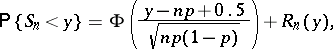the remainder term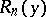has order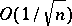uniformly for all real. For uniform approximation of the binomial distribution by means of the normal distribution the formula of Ya. Uspenskii (1937) is more useful: If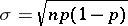, then for anyand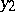,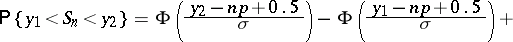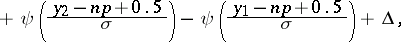where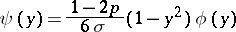and for,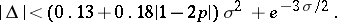To improve the relative accuracy of the approximation S.N. Bernstein [S.N. Bernshtein] (1943) and W. Feller (1945) suggested other formulas.

How to Cite This Entry:
Laplace theorem. Encyclopedia of Mathematics. URL: http://encyclopediaofmath.org/index.php?title=Laplace_theorem&oldid=12520
This article was adapted from an original article by A.V. Prokhorov (originator), which appeared in Encyclopedia of Mathematics - ISBN 1402006098. See original article